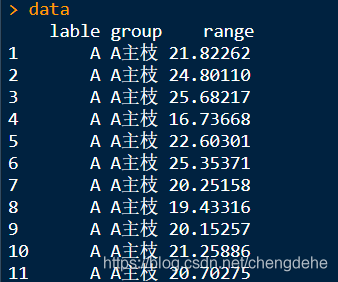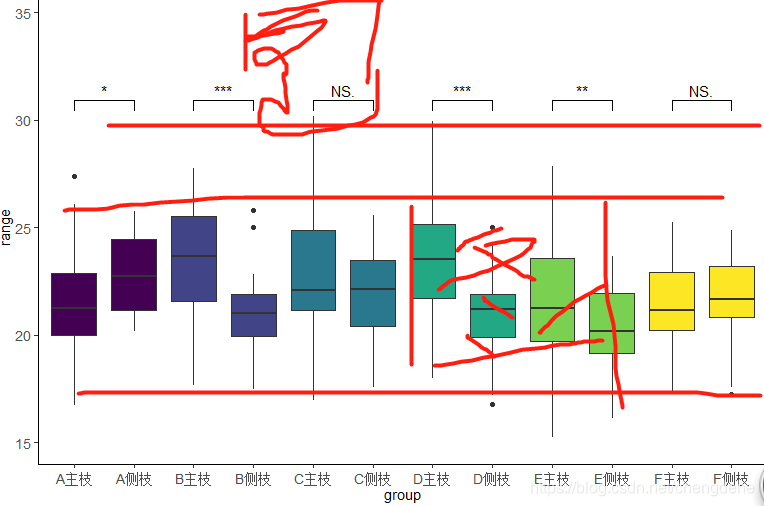# geom_ Warning message: sign function’s own error

was made by two mistakes, one day —- in memory of myself, stupid, angry to death

## the first stupid mistake is

``````**Computation failed in `stat_signif()`:not enough 'y' observations**

``````

this tells “Y” that’s not right,,,,,, this error is the most stupid
this error is looking for different,,,,,,
this is my error, maybe the same error but the error is different

``````compaired <- list(c("A主枝","A侧枝"),
c("B主枝","B侧枝"),
c("C主枝","C测枝"),
c("D主枝","D侧枝"),
c("E主枝","E侧枝"),
c("F主枝","F侧枝"))
``````

## second error

``````Warning message:Removed 12 rows containing missing values (geom_signif).

``````

and the reason why I made this error is because I wrote the y range wrong, so all the y values are in this range, but when you set the comparison,

``````geom_signif(comparisons = compaired,map_signif_level = T,test =t.test,step_increase = 0.0)
``````

step_increase = 0.0 when it is not 0, it will also occupy a certain range of Y-axis, so y needs to be expanded

**

## the third – — geom_signif function will not draw the histogram difference analysis directly between groups

**
— of course, the current understanding is not possible, I hope to know to tell you, of course, if you use the function after adding significance is possible, draw directly, like,,,,,
so I chose the boxplot, it is also good

** purpose: ** to compare whether there is a significant difference between the two data under each LABLE
LABLE

``````##install.packages("ggplot2")
library("ggplot2")
##install.packages("ggsignif")
library(ggsignif)
##读取数据
dim(data)
data[1:5,1:3]
``````lable is divided into five classes group which is divided into two classes, to perform the difference significant comparison among each A, B, C and Ddata type conversion

``````###转化因子
data\$group <-factor(data\$group,levels = unique(data\$group),ordered = T)
data\$lable <-factor(data\$lable,levels = unique(data\$lable),ordered = T)
##转换数值
data\$range <- as.numeric(data\$range)
``````

division of the comparison group

``````compaired <- list(c("A主枝","A侧枝"),
c("B主枝","B侧枝"),
c("C主枝","C测枝"),
c("D主枝","D侧枝"),
c("E主枝","E侧枝"),
c("F主枝","F侧枝"))
``````

was just used here, comparison of main and measured branches under different lable

+ A, B is for better comparison

Draw

``````p <- ggplot(data, aes(group, range,fill =lable)) +
###设置坐标，和分组
geom_boxplot(show.legend = FALSE) +
###show.legend = FALSE  去除图例
ylim(10, 40) +
##设置坐标轴Y的范围
geom_signif(comparisons = compaired,map_signif_level = T,test =t.test,step_increase = 0.0)+
##geom_signif标注显著性——具体含义可以百度此函数
## wilcox.test——两种T测验根据正太检验决定
theme_classic()+
theme(axis.text.x=element_text(angle = 0,vjust =0.5,size=10.5),axis.text.y=element_text(size=10.5))+
theme(axis.title.x =element_text(size=10.5), axis.title.y=element_text(size=10.5))
###设置刻度和标签字体等
p
``````